Stahler's (1983) Rotationally Flattened Isothermal Configurations

Consider the collapse of an isothermal cloud (characterized by isothermal sound speed,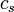$~c_s$) that is initially spherical, uniform in density, uniformly rotating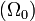$~(\Omega_0)$, and embedded in a tenuous intercloud medium of pressure,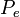$~P_e$. Now suppose that the cloud maintains perfect axisymmetry as it collapses and that$~c_s$ never changes at any fluid element. To what equilibrium state will this cloud collapse if the specific angular momentum of every fluid element is conserved? In a paper titled, The Equilibria of Rotating, Isothermal Clouds. I. - Method of Solution, S. W. Stahler (1983a, ApJ, 268, 155 - 184) describes a numerical scheme — a self-consistent-field technique — that he used to construct such equilibrium states.

In what follows, lines of text that appear in a dark green font have been extracted verbatim from Stahler (1983a).

Governing Equations

Stahler (1983a) states that the equilibrium configuration is found by solving the equation for momentum balance together with Poisson's equation for the gravitational potential,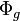$~\Phi_g$. Stahler chooses to use the integral form of Poisson's equation to define the gravitational potential, namely (see his equation 10, but note the sign change and "pink comment" shown here on the right),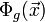$~ \Phi_g(\vec{x})$$~=$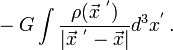$~ - G \int \frac{\rho(\vec{x}^{~'})}{|\vec{x}^{~'} - \vec{x}|} d^3x^' \, .$

As is clear from our separate discussion of the origin of Poisson's equation, this matches the expression for the scalar gravitational potential that is widely used in astrophysics.

Working in cylindrical coordinates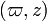$~(\varpi, z)$ — as we have explained elsewhere, the assumption of axisymmetry eliminates the azimuthal angle — Stahler states that the momentum equation is (see his equation 2):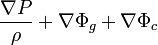$~\frac{\nabla P}{\rho} + \nabla\Phi_g + \nabla\Phi_c$$~=$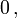$~0 \, ,$

where,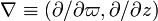$~\nabla \equiv (\partial/\partial\varpi, \partial/\partial z)$, and the centrifugal potential is given by (see Stahler's equation 3, but note the sign change and "pink comment" shown here on the right):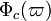$~\Phi_c(\varpi)$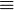$~\equiv$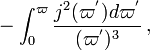$~- \int_0^\varpi \frac{j^2(\varpi^') d\varpi^'}{(\varpi^')^3} \, ,$

where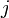$~j$ is the z-component of the angular momentum per unit mass. This last expression is precisely the same expression for the centrifugal potential that we have defined in the context of our discussion of simple rotation profiles. As Stahler stresses, by adopting a centrifugal potential of this form, he is implicitly assuming that$~j$ is not a function of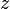$~z$; this builds in the physical constraint enunciated by the Poincaré-Wavre theorem, which guarantees that rotational velocity is constant on cylinders for the equilibrium of any barotropic fluid.

As we have demonstrated in our overview discussion of axisymmetric configurations, the equations that govern the equilibrium properties of axisymmetric structures are,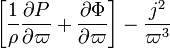$~ \biggl[ \frac{1}{\rho}\frac{\partial P}{\partial\varpi} + \frac{\partial \Phi}{\partial\varpi}\biggr] - \frac{j^2}{\varpi^3}$$~=$$~0 \, ,$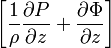$~ \biggl[ \frac{1}{\rho}\frac{\partial P}{\partial z} + \frac{\partial \Phi}{\partial z} \biggr]$$~=$$~0 \, ,$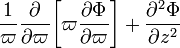$~ \frac{1}{\varpi} \frac{\partial }{\partial\varpi} \biggl[ \varpi \frac{\partial \Phi}{\partial\varpi} \biggr] + \frac{\partial^2 \Phi}{\partial z^2}$$~=$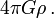$~4\pi G \rho \, .$

Let's compare this set of governing equations with the ones used by Stahler (1983a).

Scalar Virial Theorem

In an accompanying chapter where global energy considerations are explored, we have followed Shu's (1992) lead and have derived what we have referred to as a,

Generalized Scalar Virial Theorem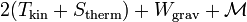$~~2 (T_\mathrm{kin} + S_\mathrm{therm}) + W_\mathrm{grav} + \mathcal{M}$$~=$$~P_e \oint \vec{x}\cdot \hat{n} dA - \oint \vec{x}\cdot \overrightarrow{T}\hat{n} dA \, .$ Shu92, p. 331, Eq. (24.12)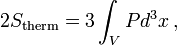$~2S_\mathrm{therm} = 3 \int_V P d^3x \, ,$

and ignoring magnetic field effects — that is, zeroing out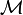$~\mathcal{M}$ and the surface integral involving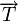$~\overrightarrow{T}$ — this generalized scalar virial theorem becomes,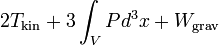$~~2 T_\mathrm{kin} + 3 \int_V P d^3x + W_\mathrm{grav}$$~=$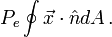$~P_e \oint \vec{x}\cdot \hat{n} dA \, .$

This exactly matches Stahler's expression for the scalar virial theorem (see his equation 16), if the external pressure,$~P_e$, is assumed to be uniform across the surface of the equilibrium configuration.

Solution Technique

Following exactly along the lines of the HSCF technique that has been described in an accompanying chapter,

Determining the Gravitational Potential

In the chapter of this H_Book that focuses on a discussion of Dyson-Wong tori, we have included the expression for the gravitational potential of a thin ring of mass,$~M$, that passes through the meridional plane at coordinate location,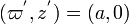$~(\varpi^', z^') = (a, 0)$, as derived, for example, by O. D. Kellogg (1929) and by W. D. MacMillan (1958; originally, 1930), namely,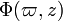$~\Phi(\varpi, z)$$~=$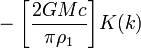$~ - \biggl[ \frac{2GMc}{\pi\rho_1}\biggr] K(k)$$~=$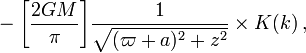$~ - \biggl[ \frac{2GM}{\pi } \biggr]\frac{1}{\sqrt{(\varpi+a)^2 + z^2}} \times K(k) \, ,$

where,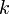$~k$$~=$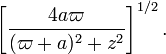$~ \biggl[ \frac{4a\varpi }{(\varpi+a)^2 + z^2} \biggr]^{1 / 2} \, .$

Stahler (1983a) has argued that a reasonably good approximation to the gravitational potential due to any extended axisymmetric mass distribution can be obtained by adding up the contributions due to many thin rings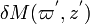$~\delta M(\varpi^', z^')$ being the appropriate differential mass contributed by each ring element — positioned at various meridional coordinate locations throughout the mass distribution. According to his independent derivation, the differential contribution to the potential,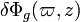$~\delta\Phi_g(\varpi, z)$, due to each differential mass element is (see his equation 11 and the explanatory text that follows it):$~\delta\Phi_g(\varpi,z)$$~=$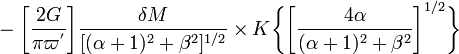$~ - \biggl[\frac{2G}{\pi \varpi^'}\biggr] \frac{\delta M}{[(\alpha + 1)^2 + \beta^2]^{1 / 2}} \times K\biggl\{ \biggl[ \frac{4\alpha}{(\alpha+1)^2 + \beta^2} \biggr]^{1 / 2} \biggr\}$$~=$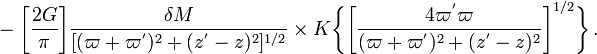$~ - \biggl[\frac{2G}{\pi }\biggr] \frac{\delta M}{[(\varpi + \varpi^')^2 + (z^' - z)^2]^{1 / 2}} \times K\biggl\{ \biggl[ \frac{4\varpi^' \varpi}{(\varpi +\varpi^')^2 + (z^' - z)^2} \biggr]^{1 / 2} \biggr\} \, .$

Stahler's expression for each thin ring contribution is clearly the same as the expressions presented by Kellogg (1929) and MacMillan (1958) for a ring that cuts through the meridional plane at$~(\varpi^', z^') = (a, 0)$.

From our broad analysis of the integral Poisson equation expressed in cylindrical coordinates, we can independently state — see the discussion of our original derivation, or separate summary — that the exact integral expression for the gravitational potential due to any axisymmetric mass-density distribution,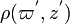$~\rho(\varpi^', z^')$, is,$~\Phi(\varpi, z)$$~=$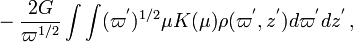$~- \frac{2G}{\varpi^{1 / 2}} \int\int (\varpi^')^{1 / 2} \mu K(\mu) \rho(\varpi^', z^') d\varpi^' dz^' \, ,$

where,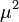$~\mu^2$$~=$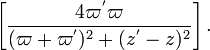$~ \biggl[\frac{4\varpi^' \varpi}{(\varpi + \varpi^')^2 + (z^' - z)^2} \biggr] \, .$

Recognizing that, for axisymmetric structures, the differential mass element is,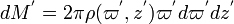$~dM^' = 2\pi \rho(\varpi^', z^') \varpi^' d\varpi^' dz^'$, this integral expression may be rewritten as,$~\Phi(\varpi, z)$$~=$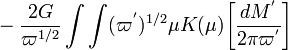$~- \frac{2G}{\varpi^{1 / 2}} \int\int (\varpi^')^{1 / 2} \mu K(\mu) \biggl[ \frac{dM^'}{2\pi \varpi^'} \biggr]$$~=$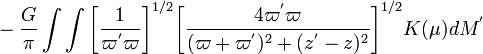$~- \frac{G}{\pi} \int\int \biggl[ \frac{1}{\varpi^'\varpi}\biggr]^{1 / 2} \biggl[\frac{4\varpi^' \varpi}{(\varpi + \varpi^')^2 + (z^' - z)^2} \biggr]^{1 / 2} K(\mu) dM^'$$~=$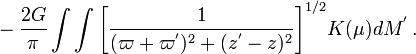$~- \frac{2G}{\pi} \int\int \biggl[\frac{1}{(\varpi + \varpi^')^2 + (z^' - z)^2} \biggr]^{1 / 2} K(\mu) dM^' \, .$

We see, therefore, that our differential contribution to the potential exactly matches Stahler's (1983a).

 © 2014 - 2021 by Joel E. Tohline |   H_Book Home   |   YouTube   | Appendices: | Equations | Variables | References | Ramblings | Images | myphys.lsu | ADS | Recommended citation:   Tohline, Joel E. (2021), The Structure, Stability, & Dynamics of Self-Gravitating Fluids, a (MediaWiki-based) Vistrails.org publication, https://www.vistrails.org/index.php/User:Tohline/citation